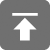# 工学1号馆

home

## 常用设计模式/容器/依赖注入/静态代理/请求对象

Wu Yudong    August 25, 2018     ThinkPHP   525

（1）为什么要使用PHP单例模式

1，php的应用主要在于数据库应用, 一个应用中会存在大量的数据库操作, 在使用面向对象的方式开发时, 如果使用单例模式,

2，如果系统中需要有一个类来全局控制某些配置信息, 那么使用单例模式可以很方便的实现. 这个可以参看zend Framework的FrontController部分。

3，在一次页面请求中, 便于进行调试, 因为所有的代码(例如数据库操作类db)都集中在一个类中, 我们可以在类中设置钩子, 输出日志，从而避免到处var_dump, echo

（2）单例模式的实现

1，私有化一个属性用于存放唯一的一个实例

2，私有化构造方法，私有化克隆方法，用来创建并只允许创建一个实例

3，公有化静态方法，用于向系统提供这个实例

(1)为什么需要工厂模式

1，工厂模式可以将对象的生产从直接new 一个对象，改成通过调用一个工厂方法生产。这样的封装，代码若需修改new的对象时，不需修改多处new语句，只需更改生产对象方法。

2，若所需实例化的对象可选择来自不同的类，可省略if-else多层判断，给工厂方法传入对应的参数，利用多态性，实例化对应的类。

<?php
/**
* 单例模式
*/
class Site {
// 属性
public $siteName; // 本类的静态实例 protected static$instance = null;
// 禁用掉构造器
private function __construct($siteName) {$this->siteName = $siteName; } // 获取本类的唯一实例 public static function getInstance($siteName = 'wuyudong') {
if (! self::$instance instanceof self) { self::$instance = new self ( $siteName ); } return self::$instance;
}
}

// 用工厂模式来生成本类的单一实例
class Factory {
// 创建指定类的实例
public static function create() {
return Site::getInstance ( "wu" );
}
}

/**
* 对象注册树
* 1.注册：set()，把对象挂到树上
* 2.获取:get（）， 把对象取下来用
* 3.注销:_unset(),把对象吃掉
*/
class Register {
// 创建对象池：数组
protected static $objects = [ ]; // 生成对象并上树 public static function set($alias, $object) { self::$objects [$alias] =$object;
}
// 从树上取下对象
public static function get($alias) { return self::$objects [$alias]; } // 把树上的对象吃掉 public static function _unset($alias) {
unset ( self::$objects [$alias] );
}
}

// 将Site类的实例上树，放进对象池
Register::set ( 'site', Factory::create () );

// 从树上取一个对象
$obj = Register::get ( 'site' ); // 查看这个对象 var_dump ($obj );
echo '<br/>';

1、任何的URL访问，最终都是定位到控制器，由控制器中某个具体的方法去执行

2、一个控制器对应一个类，如果这些类进行统一管理，怎么办？

URL访问：通过get方式将数据传到控制器指定的方法中（只能穿字符串、数值）

<?php
namespace app\common;
class Temp {
private $name; public function __construct($name = 'wuyudong') {
$this->name =$name;
}
public function setName($name) {$this->name = $name; } public function getName() { return '方法是:' . __METHOD__.'属性是：'.$this->name;
}
}

<?php
namespace app\index\controller;
use app\common\Temp;

class Demo1 {
// 通过字符串、数值用GET方式传值到类方法中
public function getName($name = 'Wu') { return 'Hello ' .$name;
}
public function getMethod1() {
$temp = new Temp ();$temp->setName ( 'hezhijuan' );
return $temp->getName (); } // app\common\Temp$temp:将会触发依赖注入
public function getMethod2(Temp $temp) {$temp->setName ( 'hezhijuan' );
return $temp->getName (); } // 绑定一个类到容器 public function bindClass1() { // 把一个类放到容器中，相当于注册到容器 \think\Container::set ( 'temp', 'app\common\Temp' ); // 将容器中的类实例化并取出来使用:实例化时可调用构造器进行初始化$temp = \think\Container::get ( 'temp', [ 'name' => 'hehehe' ] );
return $temp->getName (); } // 绑定一个类到容器（使用助手函数:bind和app） public function bindClass2() { // 把一个类放到容器中，相当于注册到容器 bind ( 'temp', 'app\common\Temp' ); // 将容器中的类实例化并取出来使用:实例化时可调用构造器进行初始化$temp = app ( 'temp', [ 'name' => 'qwqw' ] );
return $temp->getName (); } // 绑定一个闭包到容器 public function bindClosure() { // 把一个闭包放到容器中 \think\Container::set ( 'demo', function ($domain) {
return 'my blog site is：' . $domain; } ); // 将容器中的闭包取出来使用:实例化时可调用构造器进行初始化$temp = \think\Container::get ( 'demo', [
'domain' => 'www.wuyudong.com'
] );

return $temp; } } Facade静态代理 门面（facade）为容器中的类提供了一个静态调用接口，相比于传统的静态方法调用， 带来了更好的可测试性和扩展 性，你可以为任何的非静态类库定义一个 facade 类。 在common目录下新建一个Test.php文件，代码如下： <?php namespace app\common; class Test { public function hello($name) {
return 'Hello ' . $name; } } 常规动态调用代码如下： namespace app\index\controller; class Demo2 { public function index($name = 'ThinkPHP') {
$test = new \app\common\Test (); return$test->hello ( $name ); } } 如果想静态调用一个动态方法，需要给当前的类绑定一个静态代理类 新建目录facade，新建代理类Test.php，代码如下： <?php namespace app\facade; use think\Facade; class Test extends Facade { protected static function getFacadeClass(){ return 'app\common\Test'; } } 这样 <?php namespace app\index\controller; use app\facade\Test; class Demo2 { public function index($name = 'ThinkPHP') {
return Test::hello('wuyudong');
}
}


<?php

}


<?php
namespace app\index\controller;

class Demo2 {
//动态绑定
public function index($name = 'ThinkPHP') { Facade::bind('app\facade\Test', 'app\common\Test'); return Test::hello('wuyudong'); } } Request请求对象 方法1：可以使用上面所学的知识来进行Request对象的静态代理 <?php namespace app\index\controller; use think\Controller; use think\facade\Request; class Demo3 extends Controller { //创建一个请求对象Request的静态代理 public function test(){ dump(Request::get()); } } 方法2：常规方式： <?php namespace app\index\controller; use think\Controller; use think\facade\Request; class Demo3 extends Controller { public function test(){$request= new \think\Request();
dump($request->get()); } } 方法3：使用依赖注入的方式： public function test(\think\Request$request){
dump($request->get()); } 方法4：使用Request类中的request属性： public function test(){ dump($this->request->get());
}

public function test(){
dump(request()->get());
}